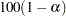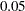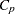Output Data Sets

### OUTEST= Data Set

The OUTEST= specification produces a TYPE=EST output SAS data set containing estimates and optional statistics from the regression models. For each BY group on each dependent variable occurring in each MODEL statement, PROC REG outputs an observation to the OUTEST= data set. The variables output to the data set are as follows:

• the BY variables, if any

• _MODEL_, a character variable containing the label of the corresponding MODEL statement, or MODELif no label is specified, whereis 1 for the first MODEL statement, 2 for the second model statement, and so on

• _TYPE_, a character variable with the value ’PARMS’ for every observation

• _DEPVAR_, the name of the dependent variable

• _RMSE_, the root mean squared error or the estimate of the standard deviation of the error term

• Intercept, the estimated intercept, unless the NOINT option is specified

• all the variables listed in any MODEL or VAR statement. Values of these variables are the estimated regression coefficients for the model. A variable that does not appear in the model corresponding to a given observation has a missing value in that observation. The dependent variable in each model is given a value of.

If you specify the COVOUT option, the covariance matrix of the estimates is output after the estimates; the _TYPE_ variable is set to the value ’COV’ and the names of the rows are identified by the character variable, _NAME_.

If you specify the TABLEOUT option, the following statistics listed by _TYPE_ are added after the estimates:

• STDERR, the standard error of the estimate

• T, thestatistic for testing if the estimate is zero

• PVALUE, the associated-value

• LB, thelower confidence limit for the estimate, whereis the nearest integer toanddefaults toor is set by using the ALPHA= option in the PROC REG or MODEL statement

• UB, theupper confidence limit for the estimate

Specifying the option ADJRSQ, AIC, BIC, CP, EDF, GMSEP, JP, MSE, PC, RSQUARE, SBC, SP, or SSE in the PROC REG or MODEL statement automatically outputs these statistics and the modelfor each model selected, regardless of the model selection method. Additional variables, in order of occurrence, are as follows:

• _IN_, the number of regressors in the model not including the intercept

• _P_, the number of parameters in the model including the intercept, if any

• _EDF_, the error degrees of freedom

• _SSE_, the error sum of squares, if the SSE option is specified

• _MSE_, the mean squared error, if the MSE option is specified

• _RSQ_, thestatistic

• _ADJRSQ_, the adjusted, if the ADJRSQ option is specified

• _CP_, thestatistic, if the CP option is specified

• _SP_, thestatistic, if the SP option is specified

• _JP_, thestatistic, if the JP option is specified

• _PC_, the PC statistic, if the PC option is specified

• _GMSEP_, the GMSEP statistic, if the GMSEP option is specified

• _AIC_, the AIC statistic, if the AIC option is specified

• _BIC_, the BIC statistic, if the BIC option is specified

• _SBC_, the SBC statistic, if the SBC option is specified

The following statements produce and display the OUTEST= data set. This example uses the population data given in the section Polynomial Regression. Figure 76.18 through Figure 76.20 show the regression equations and the resulting OUTEST= data set.

```proc reg data=USPopulation outest=est;
m1: model Population=Year;
m2: model Population=Year YearSq;
proc print data=est;
run;
```

Figure 76.18 Regression Output for Model M1
The REG Procedure
Model: m1
Dependent Variable: Population

Analysis of Variance
Source DF Sum of
Squares
Mean
Square
F Value Pr > F
Model 1 146869 146869 228.92 <.0001
Error 20 12832 641.58160
Corrected Total 21 159700

 Root MSE R-Square 25.3295 0.9197 94.648 0.9156 26.7617

Parameter Estimates
Variable DF Parameter
Estimate
Standard
Error
t Value Pr > |t|
Intercept 1 -2345.85498 161.39279 -14.54 <.0001
Year 1 1.28786 0.08512 15.13 <.0001

Figure 76.19 Regression Output for Model M2
Analysis of Variance
Source DF Sum of
Squares
Mean
Square
F Value Pr > F
Model 2 159529 79765 8864.19 <.0001
Error 19 170.97193 8.99852
Corrected Total 21 159700

 Root MSE R-Square 2.99975 0.9989 94.648 0.9988 3.16938

Parameter Estimates
Variable DF Parameter
Estimate
Standard
Error
t Value Pr > |t|
Intercept 1 21631 639.50181 33.82 <.0001
Year 1 -24.04581 0.67547 -35.60 <.0001
YearSq 1 0.00668 0.00017820 37.51 <.0001

Figure 76.20 OUTEST= Data Set
Obs _MODEL_ _TYPE_ _DEPVAR_ _RMSE_ Intercept Year Population YearSq
1 m1 PARMS Population 25.3295 -2345.85 1.2879 -1 .
2 m2 PARMS Population 2.9998 21630.89 -24.0458 -1 .006684346

The following modification of the previous example uses the TABLEOUT and ALPHA= options to obtain additional information in the OUTEST= data set:

```
proc reg data=USPopulation outest=est tableout alpha=0.1;
m1: model Population=Year/noprint;
m2: model Population=Year YearSq/noprint;
proc print data=est;
run;
```

Notice that the TABLEOUT option causes standard errors,statistics,-values, and confidence limits for the estimates to be added to the OUTEST= data set. Also note that the ALPHA= option is used to set the confidence level at 90%. The OUTEST= data set is shown in Figure 76.21.

Figure 76.21 The OUTEST= Data Set When TABLEOUT Is Specified
Obs _MODEL_ _TYPE_ _DEPVAR_ _RMSE_ Intercept Year Population YearSq
1 m1 PARMS Population 25.3295 -2345.85 1.2879 -1 .
2 m1 STDERR Population 25.3295 161.39 0.0851 . .
3 m1 T Population 25.3295 -14.54 15.1300 . .
4 m1 PVALUE Population 25.3295 0.00 0.0000 . .
5 m1 L90B Population 25.3295 -2624.21 1.1411 . .
6 m1 U90B Population 25.3295 -2067.50 1.4347 . .
7 m2 PARMS Population 2.9998 21630.89 -24.0458 -1 0.0067
8 m2 STDERR Population 2.9998 639.50 0.6755 . 0.0002
9 m2 T Population 2.9998 33.82 -35.5988 . 37.5096
10 m2 PVALUE Population 2.9998 0.00 0.0000 . 0.0000
11 m2 L90B Population 2.9998 20525.11 -25.2138 . 0.0064
12 m2 U90B Population 2.9998 22736.68 -22.8778 . 0.0070

A slightly different OUTEST= data set is created when you use the RSQUARE selection method. The following statements request only the "best" model for each subset size but ask for a variety of model selection statistics, as well as the estimated regression coefficients. An OUTEST= data set is created and displayed. See Figure 76.22 and Figure 76.23 for the results.

```proc reg data=fitness outest=est;
model Oxygen=Age Weight RunTime RunPulse RestPulse MaxPulse
/ selection=rsquare mse jp gmsep cp aic bic sbc b best=1;
proc print data=est;
run;
```

Figure 76.22 PROC REG Output for Physical Fitness Data: Best Models
The REG Procedure
Model: MODEL1
Dependent Variable: Oxygen

R-Square Selection Method

Number in
Model
R-Square C(p) AIC BIC Estimated MSE
of Prediction
J(p) MSE SBC Parameter Estimates
Intercept Age Weight RunTime RunPulse RestPulse MaxPulse
1 0.7434 13.6988 64.5341 65.4673 8.0546 8.0199 7.53384 67.40210 82.42177 . . -3.31056 . . .
2 0.7642 12.3894 63.9050 64.8212 7.9478 7.8621 7.16842 68.20695 88.46229 -0.15037 . -3.20395 . . .
3 0.8111 6.9596 59.0373 61.3127 6.8583 6.7253 5.95669 64.77326 111.71806 -0.25640 . -2.82538 -0.13091 . .
4 0.8368 4.8800 56.4995 60.3996 6.3984 6.2053 5.34346 63.66941 98.14789 -0.19773 . -2.76758 -0.34811 . 0.27051
5 0.8480 5.1063 56.2986 61.5667 6.4565 6.1782 5.17634 64.90250 102.20428 -0.21962 -0.07230 -2.68252 -0.37340 . 0.30491
6 0.8487 7.0000 58.1616 64.0748 6.9870 6.5804 5.36825 68.19952 102.93448 -0.22697 -0.07418 -2.62865 -0.36963 -0.02153 0.30322

Figure 76.23 PROC PRINT Output for Physical Fitness Data: OUTEST= Data Set
Obs _MODEL_ _TYPE_ _DEPVAR_ _RMSE_ Intercept Age Weight RunTime RunPulse RestPulse MaxPulse Oxygen _IN_ _P_ _EDF_ _MSE_ _RSQ_ _CP_ _JP_ _GMSEP_ _AIC_ _BIC_ _SBC_
1 MODEL1 PARMS Oxygen 2.74478 82.422 . . -3.31056 . . . -1 1 2 29 7.53384 0.74338 13.6988 8.01990 8.05462 64.5341 65.4673 67.4021
2 MODEL1 PARMS Oxygen 2.67739 88.462 -0.15037 . -3.20395 . . . -1 2 3 28 7.16842 0.76425 12.3894 7.86214 7.94778 63.9050 64.8212 68.2069
3 MODEL1 PARMS Oxygen 2.44063 111.718 -0.25640 . -2.82538 -0.13091 . . -1 3 4 27 5.95669 0.81109 6.9596 6.72530 6.85833 59.0373 61.3127 64.7733
4 MODEL1 PARMS Oxygen 2.31159 98.148 -0.19773 . -2.76758 -0.34811 . 0.27051 -1 4 5 26 5.34346 0.83682 4.8800 6.20531 6.39837 56.4995 60.3996 63.6694
5 MODEL1 PARMS Oxygen 2.27516 102.204 -0.21962 -0.072302 -2.68252 -0.37340 . 0.30491 -1 5 6 25 5.17634 0.84800 5.1063 6.17821 6.45651 56.2986 61.5667 64.9025
6 MODEL1 PARMS Oxygen 2.31695 102.934 -0.22697 -0.074177 -2.62865 -0.36963 -0.021534 0.30322 -1 6 7 24 5.36825 0.84867 7.0000 6.58043 6.98700 58.1616 64.0748 68.1995

### OUTSSCP= Data Sets

The OUTSSCP= option produces a TYPE=SSCP output SAS data set containing sums of squares and crossproducts. A special row (observation) and column (variable) of the matrix called Intercept contain the number of observations and sums. Observations are identified by the character variable _NAME_. The data set contains all variables used in MODEL statements. You can specify additional variables that you want included in the crossproducts matrix with a VAR statement.

The SSCP data set is used when a large number of observations are explored in many different runs. The SSCP data set can be saved and used for subsequent runs, which are much less expensive since PROC REG never reads the original data again. If you run PROC REG once to create only a SSCP data set, you should list all the variables that you might need in a VAR statement or include all the variables that you might need in a MODEL statement.

The following statements use the fitness data from Example 76.2 to produce an output data set with the OUTSSCP= option. The resulting output is shown in Figure 76.24.

```proc reg data=fitness outsscp=sscp;
var Oxygen RunTime Age Weight RestPulse RunPulse MaxPulse;
proc print data=sscp;
run;
```

Since a model is not fit to the data and since the only request is to create the SSCP data set, a MODEL statement is not required in this example. However, since the MODEL statement is not used, the VAR statement is required.

Figure 76.24 SSCP Data Set Created with OUTSSCP= Option: REG Procedure
Obs _TYPE_ _NAME_ Intercept Oxygen RunTime Age Weight RestPulse RunPulse MaxPulse
1 SSCP Intercept 31.00 1468.65 328.17 1478.00 2400.78 1657.00 5259.00 5387.00
2 SSCP Oxygen 1468.65 70429.86 15356.14 69767.75 113522.26 78015.41 248497.31 254866.75
3 SSCP RunTime 328.17 15356.14 3531.80 15687.24 25464.71 17684.05 55806.29 57113.72
4 SSCP Age 1478.00 69767.75 15687.24 71282.00 114158.90 78806.00 250194.00 256218.00
5 SSCP Weight 2400.78 113522.26 25464.71 114158.90 188008.20 128409.28 407745.67 417764.62
6 SSCP RestPulse 1657.00 78015.41 17684.05 78806.00 128409.28 90311.00 281928.00 288583.00
7 SSCP RunPulse 5259.00 248497.31 55806.29 250194.00 407745.67 281928.00 895317.00 916499.00
8 SSCP MaxPulse 5387.00 254866.75 57113.72 256218.00 417764.62 288583.00 916499.00 938641.00
9 N   31.00 31.00 31.00 31.00 31.00 31.00 31.00 31.00